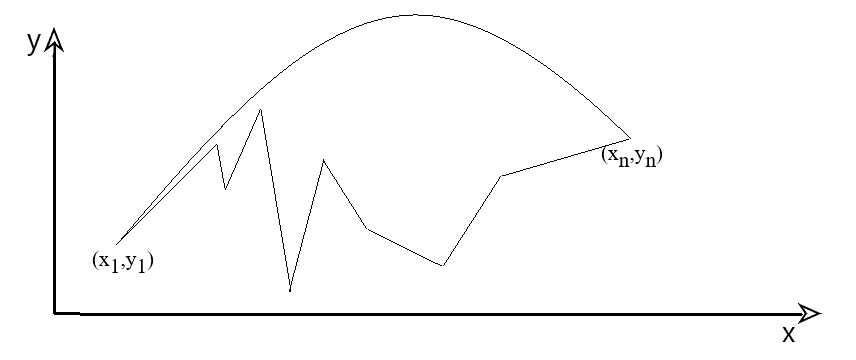시간 제한메모리 제한제출정답맞힌 사람정답 비율
1 초 (추가 시간 없음) 256 MB111100.000%

## 문제

Father Theodore, in possession of a piece of stolen sausage, has hidden in the mountains; the concessioners have got nothing else to do but try to strike him in the eye with a rock, just like the elders have always advised.

Ostap and Kisa are at the foot of the mountains; they are throwing a rock, aiming at the thief's eye. Your task is to find the minimal initial speed such that the rock will reach Father Theodore, avoiding all obstacles during its flight.For simplicity, assume that the landscape, where the three are situated, is a polyline on a plane. The coordinate $y$ for the plane denotes height. The sizes of the people are negligible, so Ostap and Kisa can be reduced to a single point on the terrain, the other point being Father Theodore's eye.

A rock flies in a parabola, subjected to gravity acceleration $g$, which, for convenience, we will assume equal to $10$. The air resistance is negligibly small. The rock must fly above the polyline.

## 입력

The first line of the input file contains the number $n$ --- the number of nodes in the polyline ($2\leq n\leq 10^5$). It is followed by $n$ lines. The $i$-th line contains two integers $x_i$, $y_i$ --- the coordinates of the $i$-th node of the polyline ($0 \leq x_i, y_i \leq 10^7$, $1 \leq i \leq n$). It is guaranteed that the sequence $x_i$ is strictly ascending, i.e. $x_i < x_j$ when $i < j$.

We know that Ostap and Kisa are in the first node of the polyline $(x_1,y_1)$, and Father Theodore is in the last node $(x_n, y_n)$.

## 출력

The output file must contain a single real number --- the minimal initial speed of the rock sufficient to give Father Theodore a black eye.

The relative or absolute error of the answer must not exceed $10^{-6}$.

## 예제 입력 1

6
1 2
4 4
5 0
6 3
8 4
11 2


## 예제 출력 1

10


## 예제 입력 2

3
0 0
1 3
5 2


## 예제 출력 2

10.4963363572